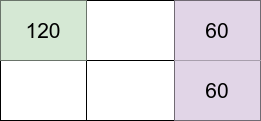##### Welcome to Subscribe On Youtube

Formatted question description: https://leetcode.ca/all/1659.html

# 1659. Maximize Grid Happiness

Hard

## Description

You are given four integers, m, n, introvertsCount, and extrovertsCount. You have an m x n grid, and there are two types of people: introverts and extroverts. There are introvertsCount introverts and extrovertsCount extroverts.

You should decide how many people you want to live in the grid and assign each of them one grid cell. Note that you do not have to have all the people living in the grid.

The happiness of each person is calculated as follows:

• Introverts start with 120 happiness and lose 30 happiness for each neighbor (introvert or extrovert).
• Extroverts start with 40 happiness and gain 20 happiness for each neighbor (introvert or extrovert).

Neighbors live in the directly adjacent cells north, east, south, and west of a person’s cell.

The grid happiness is the sum of each person’s happiness. Return the maximum possible grid happiness.

Example 1:Input: m = 2, n = 3, introvertsCount = 1, extrovertsCount = 2

Output: 240

Explanation: Assume the grid is 1-indexed with coordinates (row, column).

We can put the introvert in cell (1,1) and put the extroverts in cells (1,3) and (2,3).

• Introvert at (1,1) happiness: 120 (starting happiness) - (0 * 30) (0 neighbors) = 120
• Extrovert at (1,3) happiness: 40 (starting happiness) + (1 * 20) (1 neighbor) = 60
• Extrovert at (2,3) happiness: 40 (starting happiness) + (1 * 20) (1 neighbor) = 60

The grid happiness is 120 + 60 + 60 = 240.

The above figure shows the grid in this example with each person’s happiness. The introvert stays in the light green cell while the extroverts live on the light purple cells.

Example 2:

Input: m = 3, n = 1, introvertsCount = 2, extrovertsCount = 1

**Output: 260

Explanation: Place the two introverts in (1,1) and (3,1) and the extrovert at (2,1).

• Introvert at (1,1) happiness: 120 (starting happiness) - (1 * 30) (1 neighbor) = 90
• Extrovert at (2,1) happiness: 40 (starting happiness) + (2 * 20) (2 neighbors) = 80
• Introvert at (3,1) happiness: 120 (starting happiness) - (1 * 30) (1 neighbor) = 90

The grid happiness is 90 + 80 + 90 = 260.

Example 3:

Input: m = 2, n = 2, introvertsCount = 4, extrovertsCount = 0

Output: 240

Constraints:

• 1 <= m, n <= 5
• 0 <= introvertsCount, extrovertsCount <= min(m * n, 6)

## Solution

Use dynamic programming with pressed states. Search the cells from top to bottom and from left to right in each row. Each time a cell is searched, it may affect the cell above it and the cell to the left of it. Therefore, each state contains the current row, the current column, the number of remaining introverts, the number of remaining extroverts, and the last state. For each cell, there are three states, which are empty, an introvert and an extrovert. Loop over all possible states and obtain the maximum grid happiness.

• class Solution {
int m;
int n;
int maxState;
int mod;
int[][][][][] dp;

public int getMaxGridHappiness(int m, int n, int introvertsCount, int extrovertsCount) {
this.m = m;
this.n = n;
maxState = (int) Math.pow(3, n);
mod = maxState / 3;
dp = new int[m][n][introvertsCount + 1][extrovertsCount + 1][maxState];
return dp(0, 0, introvertsCount, extrovertsCount, 0);
}

public int dp(int row, int column, int introvertsCount, int extrovertsCount, int lastState) {
if (row == m)
return 0;
if (column == n)
return dp(row + 1, 0, introvertsCount, extrovertsCount, lastState);
if (dp[row][column][introvertsCount][extrovertsCount][lastState] == 0) {
int value = dp(row, column + 1, introvertsCount, extrovertsCount, lastState % mod * 3);
if (introvertsCount != 0) {
int value1 = 120, up = lastState / mod, left = lastState % 3;
if (row > 0 && up != 0) {
value1 -= 30;
value1 += up == 1 ? -30 : 20;
}
if (column > 0 && left != 0) {
value1 -= 30;
value1 += left == 1 ? -30 : 20;
}
value = Math.max(value, value1 + dp(row, column + 1, introvertsCount - 1, extrovertsCount, lastState % mod * 3 + 1));
}
if (extrovertsCount != 0) {
int value2 = 40, up = lastState / mod, left = lastState % 3;
if (row > 0 && up != 0) {
value2 += 20;
value2 += up == 1 ? -30 : 20;
}
if (column > 0 && left != 0) {
value2 += 20;
value2 += left == 1 ? -30 : 20;
}
value = Math.max(value, value2 + dp(row, column + 1, introvertsCount, extrovertsCount - 1, lastState % mod * 3 + 2));
}
dp[row][column][introvertsCount][extrovertsCount][lastState] = value;
}
return dp[row][column][introvertsCount][extrovertsCount][lastState];
}
}